# CREST Reasoning Olympiad Class 8 Sample Papers

## Syllabus:

Section 1: Series Completion and Inserting the Missing Character, Analogy and Classification, Blood Relations, Coding-Decoding, Direction Sense Test, Logical Venn Diagram, Alpha Numeric Sequence Puzzle, Number, Ranking and Time Sequence Test, Logical Sequence of Words, Alphabet Test, Mathematical Operations, Analytical Reasoning, Mirror Images and Water Images, Embedded Figures, Figure Formation, Construction of Squares, Grouping of Identical Figures, Figure Matrix, Paper Folding and Paper Cutting, Cubes and Dice, Dot Situations.

Achievers Section: Higher Order Thinking Questions - Syllabus as per Section 1

 Q.1 Select the figure which satisfies the same conditions of placement of the dots as in Figure-X.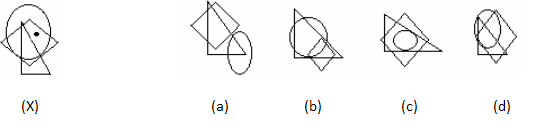Q.2 Select a suitable figure from the alternatives that would complete the figure matrix.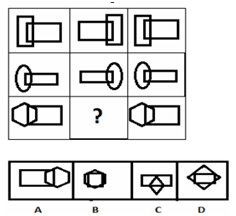Q.3 Which element will be fifth to the right of ninth from the right end if all the numbers are removed from the above arrangement? 5DGE«79\$F16R%LIAJ3B#4@KP8UM 2
 Q.4 Observe the dots on a dice (one to six dots) in the following figures. How many dots are contained on the face opposite to that containing four dots?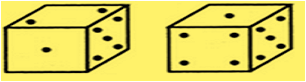Q.5 Select a suitable figure from the four alternatives that would complete the figure matrix.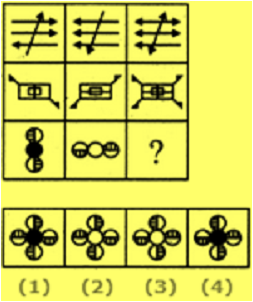Q.6 Find out from amongst the four alternatives as to how the pattern would appear when the transparent sheet is folded at the dotted line.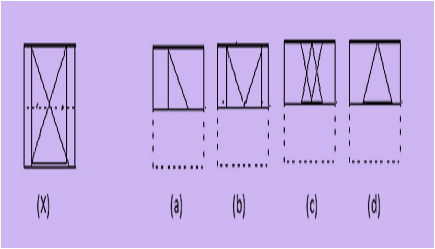Q.7 There is a Cube whose dimensions are 4 x 4 x 4 cm.Now the cube is cut into small cubes of side 1 cm. How many small cubes have three faces coloured ?
 Q.8 Select the figure which satisfies the same conditions of placement of the dots as in Figure-X.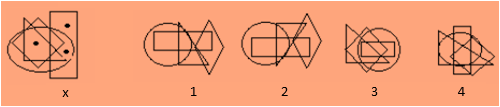Q.9 If it is possible to make one meaningful word with the first, fourth, fifth, seventh letters of the word NURSING, using each letter only once, then which of the following will be the third of that word?
 Q.10 Choose a figure which would most closely resemble the unfolded form of Figure.Sample PDF of CREST Reasoning Olympiad for Class 8: